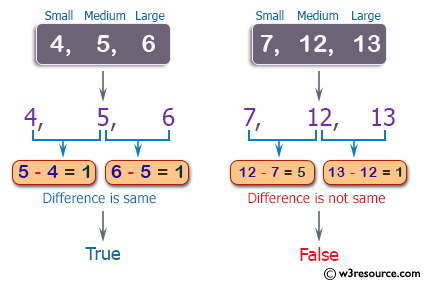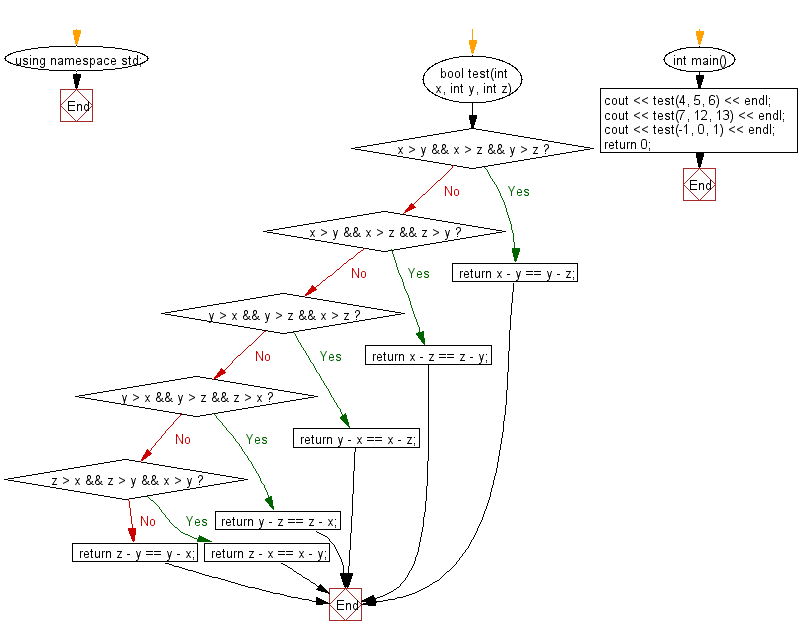﻿ C++ : Difference of small, medium and medium, large values# C++ Exercises: Check three given integers and return true if the difference between small and medium and the difference between medium and large is same

## C++ Basic Algorithm: Exercise-56 with Solution

Write a C++ program to check three given integers (small, medium and large) and return true if the difference between small and medium and the difference between medium and large is same.

Sample Solution:

C++ Code :

``````#include <iostream>

using namespace std;

bool test(int x, int y, int z)
{
if (x > y && x > z && y > z) return x - y == y - z;
if (x > y && x > z && z > y) return x - z == z - y;
if (y > x && y > z && x > z) return y - x == x - z;
if (y > x && y > z && z > x) return y - z == z - x;
if (z > x && z > y && x > y) return z - x == x - y;
return z - y == y - x;
}

int main()
{
cout << test(4, 5, 6) << endl;
cout << test(7, 12, 13) << endl;
cout << test(-1, 0, 1) << endl;
return 0;
}
``````

Sample Output:

```1
0
1
```

Pictorial Presentation:Flowchart:C++ Code Editor: